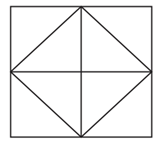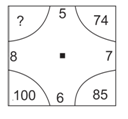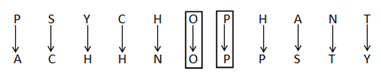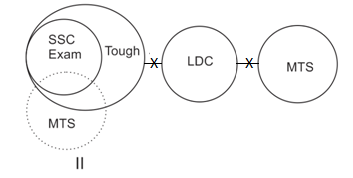# SSC CGL EXAMS 2019 | Reasoning Ability Practice Questions (Day-23)

Dear Aspirants, Here we have given the Important SSC Exam 2019 Practice Test Papers. Candidates those who are preparing for SSC 2019 can practice these questions to get more confidence to Crack SSC 2019 Examination.

[WpProQuiz 4986]

1)  ‘Statement and Conclusion’ is related to ‘Logical reasoning’, in the same way ‘Dice’ is related to?

a) Non- Verbal Reasoning

b) Verbal Reasoning

c) Analytical Reasoning

d) Decision Malang

2) Find the next term of the given series—

51, 52, 48, 57, 41, ?

a) 56

b) 66

c) 36

d) 63

3) How many such letters are there is the word ‘PSYCHOPHANT’ each of which is as far away from the beginning of the word as when they are arranged in alphabetical order?

a) None

b) One

c) Three

d) Two

4) In the following question, find the odd numbers?

a) 368149

b) 153609

c) 266404

d) 254952

5) Book is written as — ‘4153’ then in the same code language ‘TABLE’ is written as–

a) 45141

b) 45541

c) 44556

d) None of these

6) Which of the following expression will be true of the expression “Router > Operator = Android > System < Table” is definitely true?

a) System < Router

b) Table < Router

c) Operator > Table

d) Table > Android

7) In the following question below are given some statements followed by some conclusions. Taking the given statements to be true even if they seem to be at variance from commonly known facts, read all the conclusions and then decide which of the given conclusion logically follows the given statements.

Statements:

1. All SSC Exams are Tough.

II No Tough is LDC.

III. No LDC is MTS

Conclusion:

1. No SSC Exam is LDC.
2. Some Tough is SSC Exams.

III. No MTS is LDC.

a) All I, II & III are true

b) Only I & II are true

c) Only I & III are true

d) None of these

8) How many triangles and squares are there in the figure given below?a) 12, 18

b) 6, 12

c) 12,6

d) 6, 6

9) Fill in the black space of the given figure.a) 98

b) 102

c) 106

d) 89

10) A clock strike 12 taking 40 seconds in order to strike 4 at the same time taken in week is?

a) 1/55440 week

b) 1/14120

c) 1/10000 week

d) 1/ 7*60*60 week

51 + 12 = 52;

52 – 22 = 48;

48 + 32 = 57;

57 – 42 = 41

∴ 41 + 52 = 66

Given Word,

PSYCHOPHANT

Alphabetically,(3 + 6) = 92 = 81 = 8 – 1 = 72 = 49

= 36 81 49

1 + 5 = 62 = 36 => 6 – 3 = 32 = 09

2 + 6 = 82 = 64 => 6 – 4 = 22 = 04

(2 + 6)2 = 72 = 49 => 9 – 4 = 52 = 25 ≠ 52 odd.

Here we odd different prime number in each letter starting from the.

B -> 2 + 11     = 13 => 1+3 = 4

O -> 15 + 13  = 28 => 2+8 = 1+0 = 1

O ->15 + 17   = 32 => 3+2 = 5

K -> 11 + 19  = 30 => 3+0 = 3

BOOK = 4153

Similarly,

T ->  20 + 11 = 31=> 3 + 1 = 4

A -> 1 + 13 = 14 => 4 + 1 = 5

B -> 2 + 17 = 19 => 1 + 9 = 10 => 1+ 0 = 1

L -> 12 + 19 = 31 => 3 + 1 = 4

E -> 5 + 23 = 28 => 2 + 8 = 10 => 1 + 0 = 1

Table = 45141

Router > Operator = Android > System < Table Operator > Table

Operator > Table (Not true)

Table > Android (Not true)

System = Operator (Not true) as

Operator > System

Table < Router

But System < Router (True)According to above Venn diagram only conclusion I & II are definitely true for both the condition of diagram.

=>3 (3 + 1) = 12 Triangles & square = 6

100 -> 82 + 62 = 64 + 36

85 -> 62 + 72 = 36 + 49

74 -> 72 + 52 = 49 + 25

So, ? -> 82 + 52 = 64 + 25 = 89

There are 11 internals when the clock strikes 12.

Time taken for 11 internals = 40 S.

∴ Time taken for 11 internals = 40/11 S.

In order to strike 4, there are 3 internals, for which the time taken = 40*3/11 = 120/11

Now, 1 week = 7 × 24 × 60 × 60

∴ 1 day …………. 1/7 × 24 × 60 × 60 सप्ताह

∴ 120/11 s ………. (1/7 × 24 × 60 × 60) * 120/11 = 1/55440 week